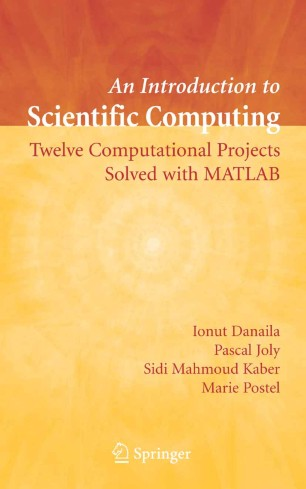# An Introduction to Scientific Computing

## Twelve Computational Projects Solved with MATLAB

• Ionut Danaila
• Pascal Joly
• Sidi Mahmoud Kaber
• Marie PostelTextbook

1. Front Matter
Pages I-XV
2. Pages 49-83
3. Pages 111-125
4. Pages 127-150
5. Pages 151-164
6. Pages 165-191
7. Pages 193-212
8. Back Matter
Pages 285-294

### Introduction

This book provides twelve computational projects aimed at numerically solving problems from a broad range of applications including Fluid Mechanics, Chemistry, Elasticity, Thermal Science, Computer Aided Design, Signal and Image Processing. For each project the reader is guided through the typical steps of scientific computing from physical and mathematical description of the problem, to numerical formulation and programming and finally to critical discussion of numerical results. Considerable emphasis is placed on practical issues of computational methods. The last section of each project contains the solutions to all proposed exercises and guides the reader in using the MATLAB scripts. The mathematical framework provides a basic foundation in the subject of numerical analysis of partial differential equations and main discretization techniques, such as finite differences, finite elements, spectral methods and wavelets).

The book is primarily intended as a graduate-level text in applied mathematics, but it may also be used by students in engineering or physical sciences. It will also be a useful reference for researchers and practicing engineers.

### Keywords

Analysis MATLAB Signal Wavelet computer-aided design (CAD) finite element method finite elements image processing kinetics mechanics model numerical analysis optimization programming scientific computing

### Editors and affiliations

• Ionut Danaila
• 1
• Pascal Joly
• 1
• Sidi Mahmoud Kaber
• 1
• Marie Postel
• 1
1. 1.Laboratoire Jacques-Louis LionsUniversité Pierre et Marie CurieParisFrance